Function Repository Resource:

# SampleUnivariateFunctions

Get one or more functions of a single complex variable

Contributed by: Paco Jain
 ResourceFunction["SampleUnivariateFunctions"][] returns a random univariate function in terms of the complex variable z, and possibly one or more parameters. ResourceFunction["SampleUnivariateFunctions"][n] returns a sample of n a random functions, for integer n. ResourceFunction["SampleUnivariateFunctions"][All] returns all available functions.

## Details and Options

By default, ResourceFunction["SampleUnivariateFunctions"] returns expressions written in terms of the independent variable z (FormalZ) and zero or more other formal symbols representing unspecified parameters. Domains in z are presumed to be the entire complex plane, but extra parameters may have additional restrictions. It is up to the user to pick suitable values for these extra parameters.
ResourceFunction["SampleUnivariateFunctions"] takes the following options:
 "IncludedSubexpressions" {} all of the supplied forms must appear as subexpressions of returned functions "ExcludedSubexpressions" {} none of the supplied forms may appear as subexpressions of returned functions "OutputForm" Automatic choose the syntactic form in which to return the functions "TrueUnivariate" Automatic whether to return only functions free of extra symbolic parameters
Possible values for "OutputForm" include Automatic, "Held", "PureFunction" and "NestedFunction".
Possible values for "TrueUnivariate" include True, False and Automatic.

## Examples

### Basic Examples (2)

Get some arbitrary sample functions:

 In:=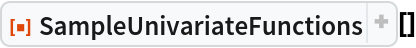Out=In:=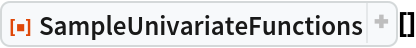Out=In:=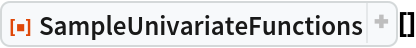Out=In:=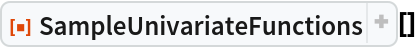Out=Get a random sample of five univariate functions:

 In:=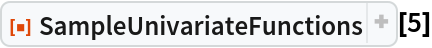Out=### Scope (2)

To get all available functions, use the argument All:

 In:=Out=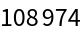Besides the independent variable z, functions may contain unspecified parameters represented by formal symbols: (here, a, b, c, d and e):

 In:=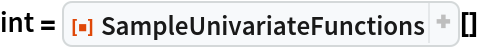Out=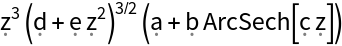Formal symbols reside in the "System`" context:

 In:=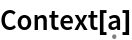Out=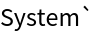They are protected and cannot be assigned values:

 In:=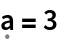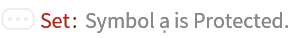Out=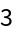Instead, use pattern replacement to give values to such symbols:

 In:=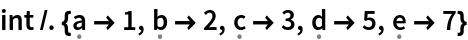Out=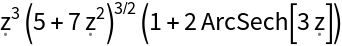### Options (12)

#### IncludedSubexpressions (3)

Get a single function containing BesselJ as a subexpression:

 In:=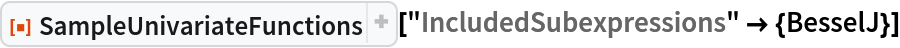Out=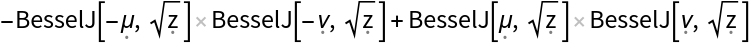Get all sample functions containing BesselJ as a subexpression and count the number available:

 In:=Out=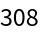View a subset of five of these functions:

 In:=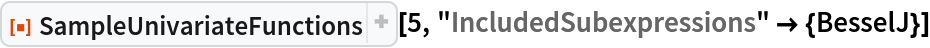Out=Every pattern listed in the value for "IncludedSubexpressions" must be separately included in the result. As such, additional forms in the list value of "IncludedSubexpressions" always reduce (or leave unchanged) the numbers of results:

 In:=Out=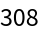In:=Out=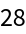The second sample is a subset of the first:

 In:=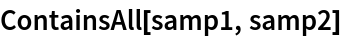Out=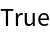To form a more inclusive pattern, allowing either BesselJ or BesselK, instead use Alternatives:

 In:=Out=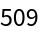#### ExcludedSubexpressions (2)

Get all sample functions containing BesselJ, but excluding Hypergeometric0F1Regularized and Sum:

 In:=Get a count of the number of such functions:

 In:=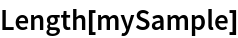Out=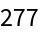#### OutputForm (4)

Results can be returned in one of several forms, as determined by the "OutputForm" option. The setting "OutputForm"Automatic (the default) returns bare expressions, written in terms of formal symbols:

 In:=Out=For ease in substituting user-supplied parameter/variable values, the setting "OutputType""PureFunction" can be useful (note that the independent variable, z, always appears as the first argument of the returned Function expressions):

 In:=Out=On supplying values, Function ignores extra parameters, using only the number needed to fill its slots:

 In:=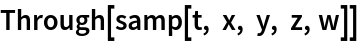Out=The setting "OutputType""NestedFunction" separates the independent variable (z) from other parameters using separate Function wrappers:

 In:=Out=The setting "OutputForm" "Held" causes results to be returned wrapped in Hold:

 In:=Out=The setting "OutputForm""PureFunction" can be preferable for certain calculations (e.g., using Derivative, Plot, …):

 In:=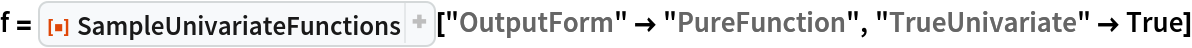Out=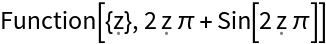In:=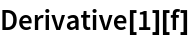Out=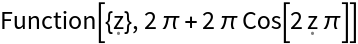In:=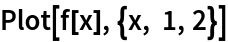Out=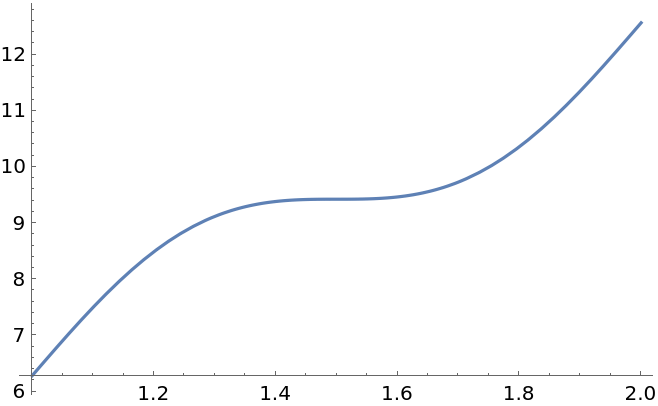Using "OutputForm" "Held" can result in quicker returns for large batches of functions (e.g., All), as each expression is shielded from kernel evaluation:

 In:=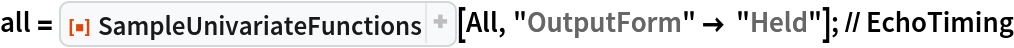0.6864938`
 In:=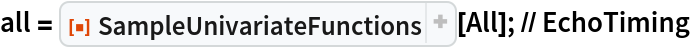107.6802886`

Auto-evaluation can cause some functions to change form, hiding the inclusion of certain subexpressions:

 In:=Out=Though we requested a function containing Sqrt as a subexpression, it is absent in the result:

 In:=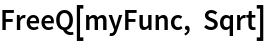Out=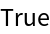By viewing the FullForm, we see that what the front end renders as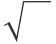is actually Power[#,Rational[1,2]& at the expression level:

 In:=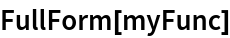Out=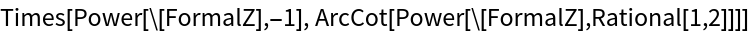Using "OutputForm" "Held" preserves the "original" form of expressions, prior to kernel evaluation:

 In:=Out=We now see the Sqrt we expect in the expression's FullForm:

 In:=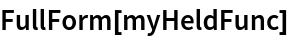Out=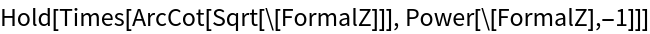#### TrueUnivariate (3)

To get functions free of any symbolic parameters besides the independent variable z, use the option setting "TrueUnivariate"True:

 In:=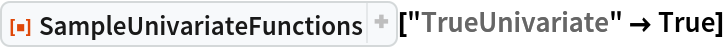Out=The setting "TrueUnivariate" False returns only functions that do contain extra parameters:

 In:=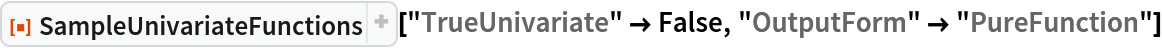Out=The default setting "TrueUnivariate" Automatic returns a mix of functions that do and do not contain extra parameters:

 In:=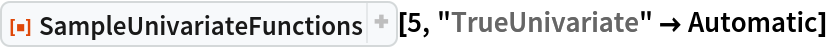Out=### Properties and Relations (1)

Use SeedRandom to get a repeatable sequence of functions:

 In:=Out=In:=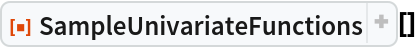Out=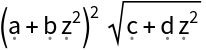In:=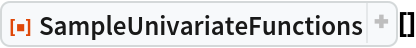Out=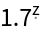### Neat Examples (2)

Create a gallery of plots of random functions and their first derivatives. First get six random functions:

 In:=Out=Partition the function list and create a grid of plots:

 In:=Out=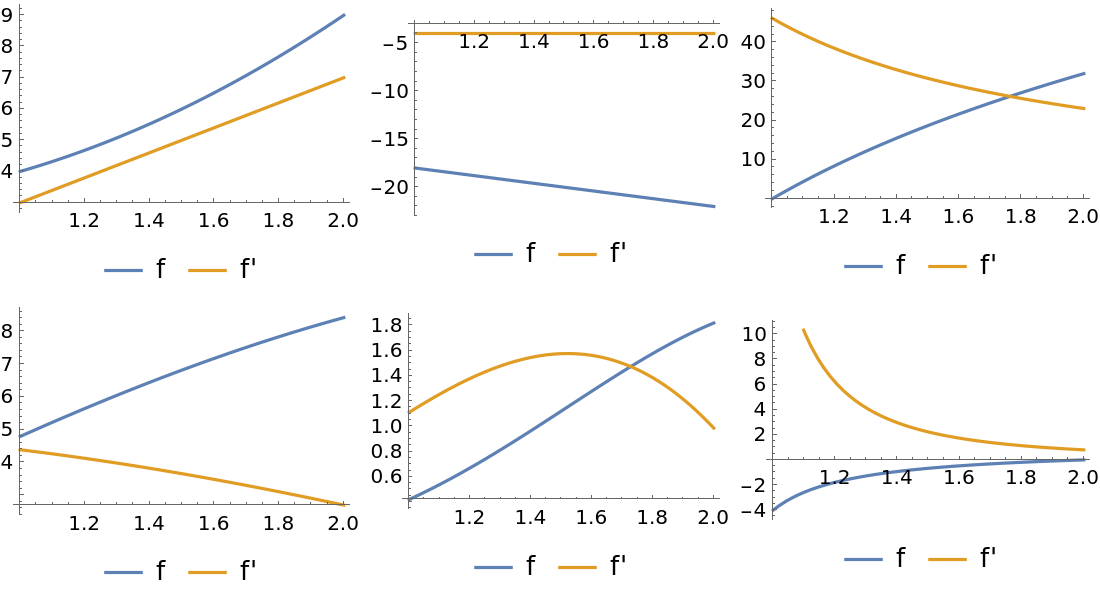## Publisher

Wolfram|Alpha Math Team

## Version History

• 1.2.1 – 17 January 2023
• 1.2.0 – 10 January 2023
• 1.1.0 – 13 December 2022
• 1.0.0 – 29 November 2022